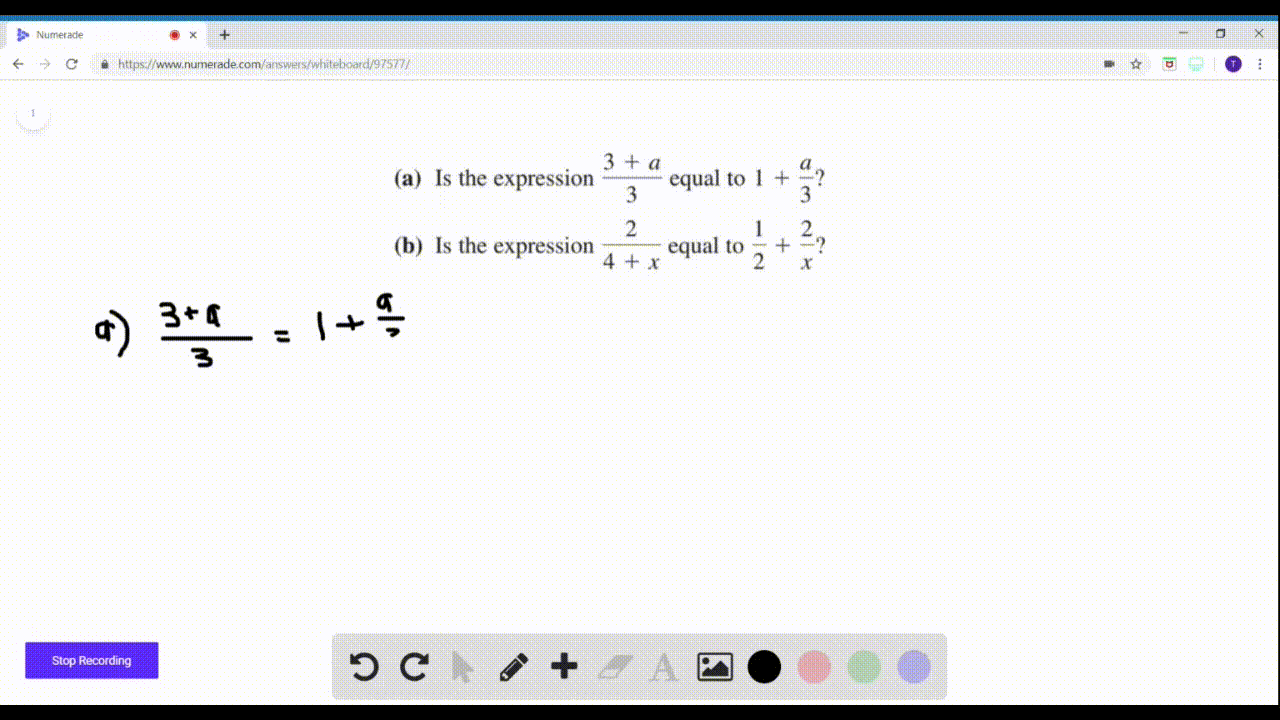Enroll in one of our FREE online STEM summer camps. Space is limited so join now!View Summer Courses### Yes or No? If $N o,$ give a reason. (Disregard an…

02:07Winsor School

Need more help? Fill out this quick form to get professional live tutoring.

Get live tutoring
Problem 5

Yes or No? If $N o,$ give a reason. (Disregard any value that makes a denominator zero.)
\begin{equation}
\begin{array}{l}{\text { (a) Is the expression } \frac{x(x+1)}{(x+1)^{2}} \text { equal to } \frac{x}{x+1} ?} \\ {\text { (b) Is the expression } \sqrt{x^{2}+25} \text { equal to } x+5 ?}\end{array}
\end{equation}

a) YES
b) $\mathrm{NO}$

## Discussion

You must be signed in to discuss.

## Video Transcript

So the first question A idiot ex Texas one another. But it was home square. We see it that there's a common experts One in the new maid ended up meeting, which means that we can cancel this out. And now we have is X divided by because this is experts one squared. They got one of one of the close ones. No, you have sex because one. So, in fact, the original expression is equal to X divided by a close one. So for a we have yes, it is true. Now for B, the question asks is the square root of X squared plus 25 equal to express Hi. So that we can do is you can ask the question square with the X squared close when you die. Cool. Perhaps the ex classified. So every square, both sides and your ex way post 25 on the other side Have a question like that You have excluded post tonight I was 25 that in fact, these are not equal because you have an extra pen x here. So the certain question answered news? No, This fault